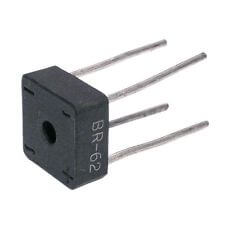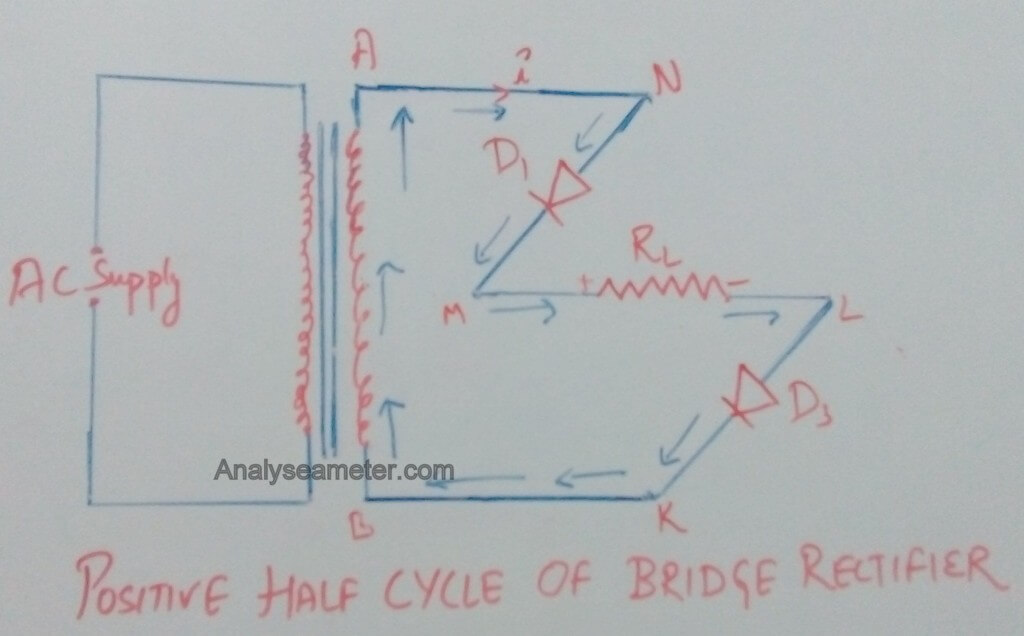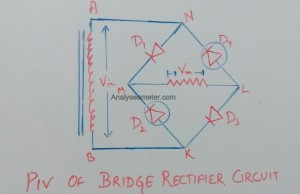# Bridge Rectifier Circuit, Operation, Characteristics & Advantages

Full wave bridge rectifier is another type of full wave rectifier used to convert an alternating current input into a direct current output. In a bridge circuit configuration, it comprises of four diodes that provide the same polarity of output for either polarity of the input. The main feature of this diode bridge which makes it more efficient than centre tap rectifier is its polarity i.e. the polarity of output is same as that of input. In our previous articles, we explain rectifier, half wave rectifier, Efficiency equation of half wave rectifier, efficiency equation of full wave rectifier etc. In this tutorial, we are going to explain bridge rectifier circuit, operation, uses, advantages & disadvantages in detail.## Bridge Rectifier

In this rectifier, we use an ordinary transformer in place of centre tap transformer which makes it more efficient. The full wave bridge rectifier circuit contains four diodes D1, D2, D3, D4 which are connected in a form of a bridge. The AC supply to be rectified is applied to the diagonally opposite ends of the bridge whereas the load resistor RL is connected across the remaining two diagonally opposite ends of the bridge. The circuit and waveform diagram of the full wave bridge rectifier is given below.## Operation of Bridge rectifier:

The operation of the full bridge rectifier is easily understood with the help of circuit diagram and waveform whose figure is given below. When AC supply is switched on, the alternating voltage Vin  starts to appear across the AB terminals of the secondary winding of the transformer which needs rectification. Diodes alternatively conduct according to the cycle of the waveform i.e positive half cycle and negative half cycle.

### During positive Half cycle:During the positive half-cycle of the input voltage, the upper end of the transformer i.e. A is positive with respect to the lower end of the transformer i.e., B. This makes the diode D1 and D3 forward biased which allows the current flow through it and enters the load resistor RL . While the diode D2 and Dare reverse biased which do not allow the current to flow through it. Thus, the direction of current flow through the diode D1 and D3 is shown in the figure below by solid arrows.

### During Negative Half cycle:During the negative half-cycle of the input voltage, the upper end of the transformer i.e. A is negative with respect to the lower end of the transformer i.e., B. This makes the diode D2 and D4 forward biased which allows the current flow through it and enters the load resistor RL . While the diode D1 and D3 are reverse biased which do not allow the current to flow through it. Thus, the direction of current flow through the diode D2 and D4 is shown in the figure below by solid arrows.

Note: During both the half cycles current flows through the load resistor RL in the same direction.

Therefore, DC output voltage Vout is obtained across the load resistor RL .

## Full wave Bridge Rectifier Characteristics:

For the analysis of bridge rectifier circuit, you must have to be considered following parameters or characteristics in mind. Following of its characteristics are:

### Peak inverse voltage

Peak inverse voltage is defined as the maximum value of the voltage coming out of the diode when it is reverse biased during the negative half cycle. For instance, when secondary voltage attains its positive maximum value & the terminal A is positive with respect to B terminal, the diode D1 and D3 are forward biased starts conducting current. Therefore, as shown in the figure below, terminal M attains the same voltage as that of terminal N and terminal L attains the same voltage as that of K indicates that the PIV across both of them is  VM.### (ii) Root mean square value of voltage and current:

RMS value is defined as the effective value of a varying voltage or current. Across the load resistor RL , the effective value of voltage and current is given as:

Irms =   ʃ (i2 dθ) / π ….. (i)

Integrate equation (i) from 0 to π,

= √ (1 / π) * ʃ Im2 sin2 θ dθ= √ (I

= √ (Im2 / π) * ʃ ( 1- cos 2θ)/ 2  dθ= √ (I

= √ (Im2 / 2π) * [ ʃ dθ – ʃ cos 2θ dθ ]= √ (I

= √ (Im2 / 2π) * [[θ] – [sin 2θ / 2]]= √ (I

= √ (Im2 / 2π) * [π – 0]

Therefore,

Irms = Im / √ 2

As we know,

Vrms = Irms * RL

Therefore, by putting the value of  Irms from the above equation it is given as:

Vrms = (Im / √ 2 * RL)

### (iii) DC output Voltage and current:

The average or DC value of voltage and current across the load resistor is given as follows:

As we know,

Idc = ʃ (i dθ) / π   ….. (ii)

Integrate equation (ii) from 0 to π,

= (1 / π) * ʃ Im sin θ dθ

= (Im / π) * ʃ sin θ dθ

= (Im / π) [ – cos θ ]

= (Im / π) [ -(-1-1)]

= 2 (Im / π)

Therefore,

Idc = (2Im / π)

As we know,

Vdc= Idc * RL

Therefore,

Vdc= (2Im / π)  * RL

Today’s in electronics for power supply circuit, we prefer bridge rectifier circuit because of its reliability and efficiency. Following of its advantages and disadvantages are:

• For high voltage applications, a bridge rectifier is preferred because it has high peak inverse voltage.
• It has high transformer utilization factor.
• It can be constructed with or without transformer i.e. it does not require centre tap transformer. If a transformer is involved, any ordinary step down/step up transformer can be used.